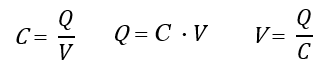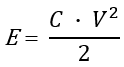# Capacitor Energy & Charge Calculator

Easily calculate the charge and energy of any capacitor given its capacitance and voltage. Supports multiple measurement units (mv, V, kV, MV, GV, mf, F, etc.) for inputs as well as output (J, kJ, MJ, Cal, kCal, eV, keV, C, kC, MC, etc.).

Share calculator:

Embed this tool:
get code

## Using the capacitor charge & energy calculator

This tool functions both as a capacitor charge calculator and a capacitor energy calculator with the required input being the same in both cases: the capacitance and voltage running through the capacitor. It supports a wide range of input and output measurement units. Simply enter the two required measurements and choose the output units for both energy and charge (or use the default).

The calculator uses well-known equations (as explained below) and conversion operations.

## Capacitor charge, energy, capacitance and voltage explained

A capacitor consists of two parallel conductive (metal) plates which are separated by special insulating material called a "dielectric". When a voltage is applied to the plates one plate is charged positively with respect to the supply voltage, while the other has an equal and opposite negative charge. This results in the unique quality of the capacitor to contain an electric charge, much like a rechargeable battery. The electrical charge a capacitor can hold is denoted by Q (measured in Coulombs (C)).

The voltage (V) is simply the difference in electric potential between two points and is measured in Volts (V). A capacitor's ability to store an electrical charge between its plates is called capacitance and is denoted with C and is measured in Farads (F) which equals 1 Coulomb/Volt. It is proportional to the size of the plates and the inversely-proportional to the distance between the plates. The energy (E) is the amount of work that the stored charge can perform and is measured in Joules, electron-Volts, Calories, etc.

## Capacitor Charge Equations

From the relations between charge (Q), capacitance (C) and voltage (V) we can express the capacity charge formula as these three equations:The first shows how to find the capacitance based on charge and voltage, the second is the capacitor charge equation while the third is the capacitor voltage equation.

## Capacitor Energy Formula

A capacitor's energy (or work) can also be calculated if its capacitance (C) and voltage (V) are known, using the equation:where E is the energy (sometimes written as W for work).## Capacitor Charge & Energy Calculation Examples

Example 1: A capacitor on a computer motherboard is known to have capacitance of 5 Farads and the voltage is known to be 50 mV. What is the capacitor's charge in Farads? Since a 1 Coulomb = 1 Farad-Volt we first convert 50 mV to 0.050 V and then apply the capacitor charge equation C = Q · V = 5 · 0.050 = 0.25 C.

Of course, while using our capacitor charge calculator you would not need to perform these unit conversions, as they are handled for you on the fly.

Example 2: A capacitor is graded at 0.1 Farads and the voltage is known to be 10 Vats. What is the capacitor's energy? We simply substitute in the capacitor's energy equation above: E = 0.1 · 102 / 2 = 10 /2 = 5 J (Joules).